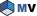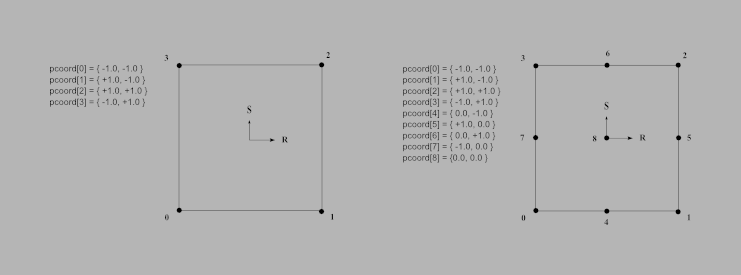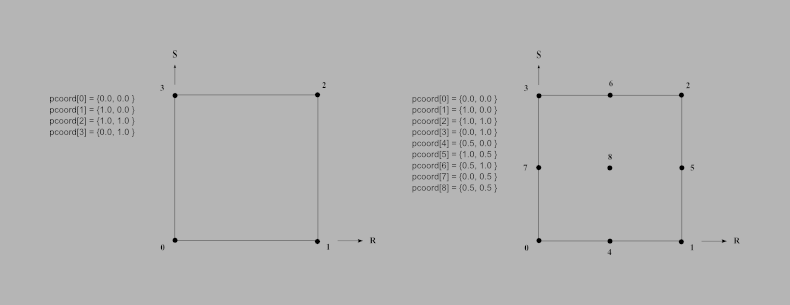# MiCell Class Reference [Cell]Defines an abstract cell of a mesh. More...

`#include <MeshVizXLM/mesh/cell/MiCell.h>`

Inheritance diagram for MiCell:List of all members.

## Public Member Functions

virtual ~MiCell ()
virtual size_t getNumNodes () const =0
virtual size_t getNodeIndex (size_t node) const =0
virtual double getRelativeSize (const MiGeometryI *meshGeometry) const
virtual void getWeight (const MbVec3d &ipcoord, std::vector< double > &weight) const
virtual MbVec3d getIsoParametricCoord (size_t nodeIndex) const
virtual MbVec3d getCenter (const MiGeometryI &geometry) const
virtual bool isPointInsideCell (const MiGeometryI &meshGeometry, const MbVec3d &point, std::vector< double > &weights) const

## Protected Member Functions

virtual std::ostream & toStream (std::ostream &s) const

## Friends

std::ostream & operator<< (std::ostream &s, const MiCell &cell)

## Detailed DescriptionDefines an abstract cell of a mesh.

A cell is a basic volume, surface or edge element that connects adjacent nodes of a mesh. The MiCell interface is necessary to define the topology of unstructured 1D, 2D or 3D meshes.

MiCell is the base class that defines topological characteristics of a cell (characteristics not depending on the cell dimension).

These methods must be overridden by the appplication as they are pure virtual. The characteristics that depend on the cell dimension are defined in the derived interfaces MiLineCell for 1D cells, MiSurfaceCell for 2D cells, and MiVolumeCell for 3D cells.

MiCell also defines additional methods with a default implementation. A default implementation is provided in 2 cases:

• A default behavior is defined. The application should override the method if this default behavior is not satisfying. getRelativeSize() is an example of such a method.
• The method throws an exception as it is only used by some extractors. When an application uses such extractors the method must be overridden. getWeight() is an example of such a method.
See also:
MiLineCell, MiSurfaceCell, MiVolumeCell
MiTopologyExplicitI, MiVolumeTopologyExplicitI, MiSurfaceTopologyExplicitI, MiLineTopologyExplicitI

## Constructor & Destructor Documentation

 virtual MiCell::~MiCell ( ) ` [inline, virtual]`

Virtual destructor.

## Member Function Documentation

 MbVec3d MiCell::getCenter ( const MiGeometryI & geometry ) const` [inline, virtual]`

Gets the center of the cell.

This method is not pure virtual because it is not used by all extraction classes. The default implementation provided computes the average of the coordinates of each cell's node.

Parameters:
 [in] geometry The geometry of the mesh. Used to retrieve the node coordinates of this cell.
Returns:
The center of the cell.
 virtual MbVec3d MiCell::getIsoParametricCoord ( size_t nodeIndex ) const` [inline, virtual]`

Gets the iso parametric coordinates of a cell's node.

Only classes defining non linear cells must implement this method.

The interval value of parametric coordinates are usually [0,1] or [-1,+1]. If the application uses interval [0,1], the parametric coordinates returned by this method for linear cell must be either 0 or 1. For quadratic cell, the parametric coordinates must be either 0, 0.5 or 1. (0.5 for intermediate nodes).

See the following image as example of parametric coordinates values for linear and quadratic quadrangle.parametric coordinates in [-1,+1]parametric coordinates in [0,1]

Note:
1D cells use only 1 parametric coordinate (MbVec3d); 2D cells use only 2 parametric coordinates (MbVec3d[0-1]); 3D cells use 3 parametric coordinates.

This method is not pure virtual because it is not used by all extraction classes. However using an extraction class that uses this method will generate an exception. An application does not need to override this method if no such extraction class is used.

Parameters:
 [in] nodeIndex The index of the node.
 virtual size_t MiCell::getNodeIndex ( size_t node ) const` [pure virtual]`

Gets the index (in the mesh) of a node.

Parameters:
 [in] node Node number (where: 0 <= node < getNumNodes()).
 virtual size_t MiCell::getNumNodes ( ) const` [pure virtual]`

Gets the number of nodes.

This number must include the additional nodes of non linear cells. For instance getNumNodes() for a quadratic triangle cell should return 6.

 virtual double MiCell::getRelativeSize ( const MiGeometryI * meshGeometry ) const` [inline, virtual]`

Gets the relative size of the cell.

This method is used only by the MoMeshCellShape to display node names if using the relative offset feature and the streamline extraction to adapt the step integration to the size of the cell. getRelativeSize() should return a value depending on the size of the cell even if the default implementation returns 1. For instance, getRelativeSize() can return the length of the longest edge of this cell, or the length of its diagonal.

If getRelativeSize() returns a constant value for any cell, the stream line extraction uses a constant number of step integration anywhere in the mesh. Returning a constant implies that all the cells are supposed to have the same size. getRelativeSize() must not return 0.

Parameters:
 [in] meshGeometry the geometry of the mesh. Used to retrieve the node coordinates of this cell.
Returns:
1 by default.
 virtual void MiCell::getWeight ( const MbVec3d & ipcoord, std::vector< double > & weight ) const` [inline, virtual]`

Gets the weights of a point defined by its iso parametric coordinates.

Only classes defining non linear cells must implement this method.

A weight value (aka shape function) must be given for each node of the cell. The weight must respect the following rules:

• The sums of the weights must be equal to 1.
• When ipcoord matches the i-th node of the cell, weight[i] must be 1 and weight[j] must be 0 for any j!=i.
• The weight depends only on pcoord for all types of 1D cells.
• The weight depends only on pcoord and pcoord for all types of 2D cells.

See chapter "Properties of shape functions" in the User's Guide for more details. This method is not pure virtual because it is not used by all extraction classes. However using an extraction class that uses this method will generate an exception. An application does not need to override this method if no such extraction class is used.

Parameters:
 [in] ipcoord iso parametric coordinates of the points where the weights must be evaluated. [out] weight Must contain at least as many elements as the number of nodes in this cell.
Note:
For extraction purpose only, it is not nessessary to resize the weight vector array since extraction classes are optimized such as weight vectors passed to this method are already allocated and large enough to retrieve all the computed weights. Thus the following assignment is then sufficient:
```  weight[i] = wi
```
for each i with 0 <= i < getNumNodes()
 virtual bool MiCell::isPointInsideCell ( const MiGeometryI & meshGeometry, const MbVec3d & point, std::vector< double > & weights ) const` [inline, virtual]`

Checks if a point is inside or outside a cell.

This method is not pure virtual because it is not used by all extraction classes. However using an extraction class that uses this method (i.e. streamline) will generate an exception. An application does not need to override this method if no such extraction class is used.

Parameters:
 [in] meshGeometry The geometry of the mesh. Used to retrieve the node coordinates of this cell. [in] point The point to be checked. [out] weights Must contain at least as many elements as the number of nodes in this cell (see getWeight()).
Returns:
True if the point is inside the cell.
Note:
For extraction purpose only, it is not nessessary to resize the weight vector array since extraction classes are optimized such as weight vectors passed to this method are already allocated and large enough to retrieve all the computed weights. Thus the following assignment is then sufficient:
```  weight[i] = wi
```
for each i with 0 <= i < getNumNodes()
 std::ostream & MiCell::toStream ( std::ostream & s ) const` [inline, protected, virtual]`

Performs an output operation on a stream which is returned afterward. This method is protected since only called by the output operator <<. This method is not pure virtual and output by default the geometry and topology of a mesh. Whenever a different output is needed, this method can be implemented in the subclass to get a different output format. No std::ostream& operator << needs to be defined in any subclass. This allows you to benefit from the virtual inheritance when using the output operator on an abstract class.

Reimplemented in MiVolumeCell.

## Friends And Related Function Documentation

 std::ostream& operator<< ( std::ostream & s, const MiCell & cell ) ` [friend]`

Outputs the mesh to a stream using the toStream protected method implemented in all derived classes.

The documentation for this class was generated from the following file:

Open Inventor Toolkit reference manual, generated on 16 Mar 2021
Copyright © Thermo Fisher Scientific All rights reserved.
http://www.openinventor.com/